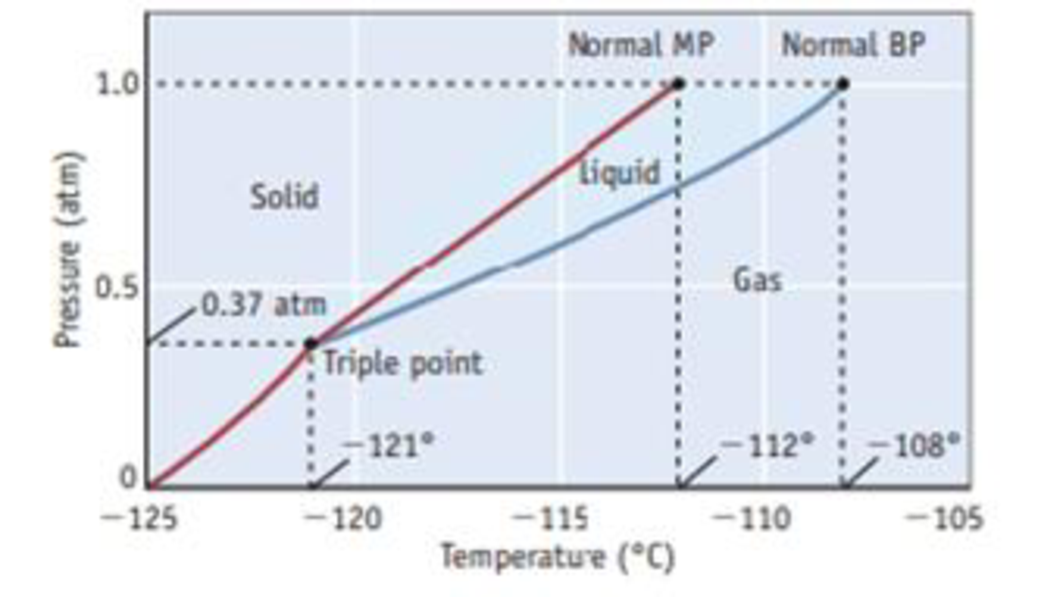Chapter 12, Problem 38PS

Chapter
Section
Textbook Problem

Use the phase diagram given here to answer the following questions:(a) In what phase is the substance found at room temperature and 1.0 atm pressure? (b) If the pressure exerted on a sample is 0.75 atm and the temperature is −114 °C, in what phase does the substance exist? (c) If you measure the vapor pressure of a liquid sample and find it to be 380 mm Hg, what is the temperature of the liquid phase? (d) What is the vapor pressure of the solid at −122 °C? (e) Which is the denser phase—solid or liquid? Explain briefly.sss

(a)

Interpretation Introduction

Interpretation:

From the phase diagram, phase that the substance found at room temperature and 1.0atm pressure has to be determined.

Concept introduction:

Under certain specific conditions, two states can coexist in equilibrium; can be represented in phase diagram.

The triple point of a substance is the temperature and pressure at which the three phases (gas, liquid, and solid) of that substance coexist in thermodynamic equilibrium.

Explanation

Phase diagram is shown here, according to the phase diagram, the compound must xenon therefore, The substance is gas phase at room temperature and one atmospheric pressure...

(b)

Interpretation Introduction

Interpretation:

From the phase diagram, phase that the substance exists has to be identified.

Concept introduction:

Under certain specific conditions, two states can coexist in equilibrium; can be represented in phase diagram.

The triple point of a substance is the temperature and pressure at which the three phases (gas, liquid, and solid) of that substance coexist in thermodynamic equilibrium.

(c)

Interpretation Introduction

Interpretation:

The temperature at which the liquid phase exists has to be determined.

Concept introduction:

Under certain specific conditions, two states can coexist in equilibrium; can be represented in phase diagram.

The triple point of a substance is the temperature and pressure at which the three phases (gas, liquid, and solid) of that substance coexist in thermodynamic equilibrium.

(d)

Interpretation Introduction

Interpretation:

According to the Phase diagram, the vapor pressure of the solid at 1220C has to be identified.

Concept introduction:

Under certain specific conditions, two states can coexist in equilibrium; can be represented in phase diagram.

The triple point of a substance is the temperature and pressure at which the three phases (gas, liquid, and solid) of that substance coexist in thermodynamic equilibrium.

(e)

Interpretation Introduction

Interpretation:

Denser phase, solid or liquid has to be determined.

Concept introduction:

Under certain specific conditions, two states can coexist in equilibrium; can be represented in phase diagram.

The triple point of a substance is the temperature and pressure at which the three phases (gas, liquid, and solid) of that substance coexist in thermodynamic equilibrium.

Still sussing out bartleby?

Check out a sample textbook solution.

See a sample solution

The Solution to Your Study Problems

Bartleby provides explanations to thousands of textbook problems written by our experts, many with advanced degrees!

Get Started